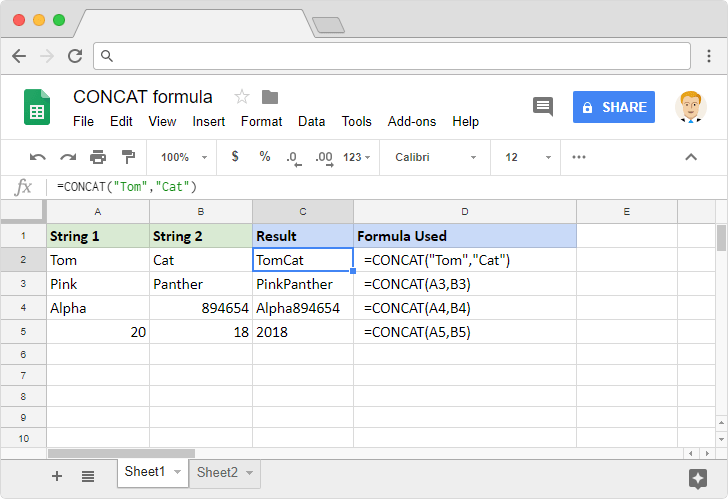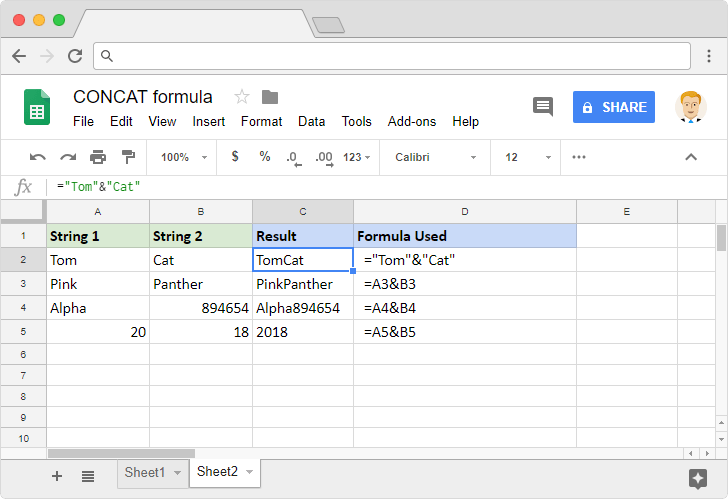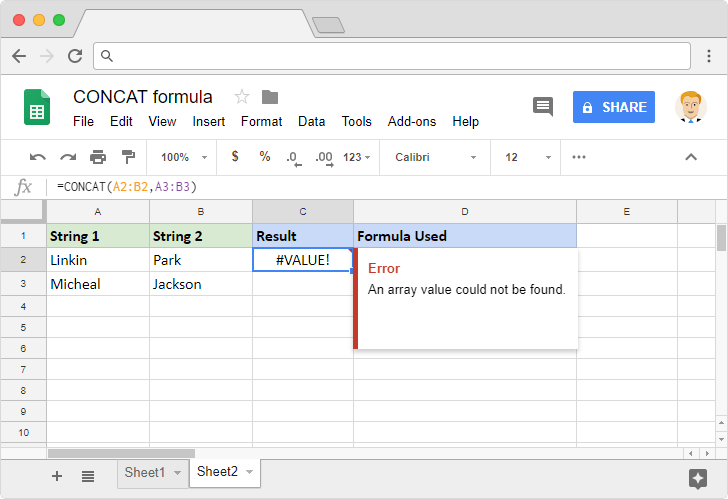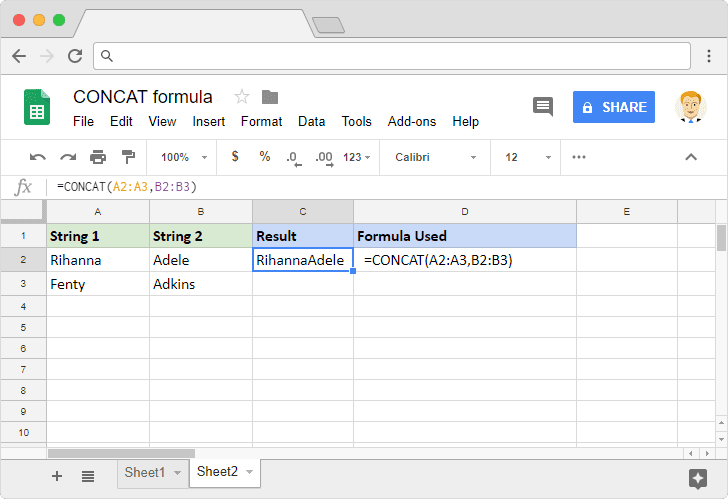# How to use the CONCAT formula in Google SheetsWhile working with strings on spreadsheets, we may have to do many operations such as trimming, extracting, and converting. What about joining two strings together? Sure, we can do that as well. The CONCAT formula in Google Sheets helps us to combine (or concatenate) two strings into one.

### Syntax

CONCAT(value1, value2)

• value1 – is the value to which the formula appends value2.
• value2 – is the value the formula appends to value1.

Please note that both value1 and value2 can be any value or reference to a value, including numeric and text types. But it isn’t a good idea to include ranges that have more than one values within them.

### Usage: CONCAT formula in Google Sheets

Let us now go ahead and look at a few examples that we tried on Google Sheets application. Please take a look at the following snapshot.Across all the examples, we’ll notice that the CONCAT formula appends the second value after the first. Regardless of whether the values are text or numeric. The functionality of this formula is the same as that of the ampersand (&) operator. We can achieve the same results using this operator. Please see the image below.There’s a limitation to the CONCAT formula – in that we can only append two values together. To that effect, it won’t give us the desired output if we supplied a range of values to its input parameters. It might result in an error as shown below.Or surprisingly, pick just the first value from each of the ranges and append them as shown below.Neither of these results is desirable when we are trying to combine values from multiple cells. Fortunately, we have a more elaborate formula called CONCATENATE. This accommodates multiple values and appends them in the sequence we provide them. Not just that, it also accepts the range of cells and combines the values from those cells.